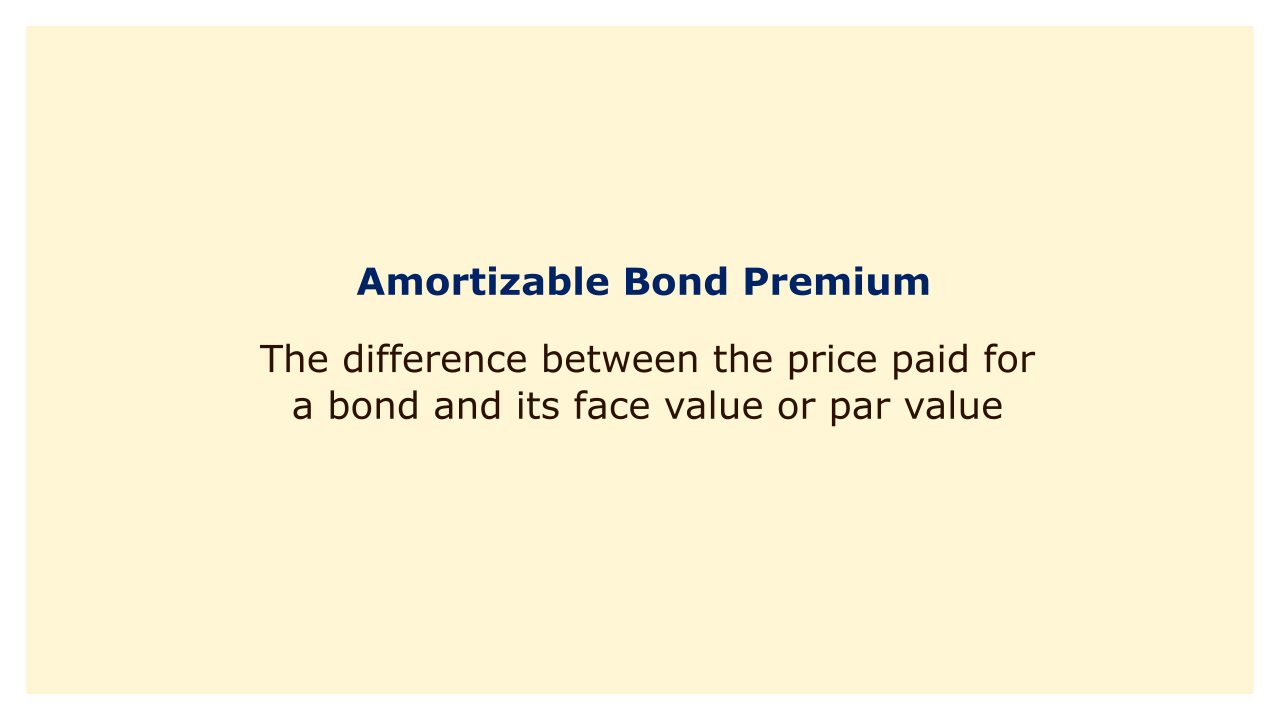Image: Moneybestpal.com

### An amortizable bond premium is the difference between the price paid for a bond and its face value or par value. It happens when a bond is sold for more than its face value, typically because the coupon rate on the bond is higher than the interest rate on the market at the time.

The bond premium is a component of the bond's cost basis and may be tax deductible if the bond earns taxable interest. The bond premium continuously decreases over the bond's lifespan until it is zero at maturity. Amortization is the name given to this process.

#### How to Calculate Amortizable Bond Premium

The constant yield method is one of the most often used approaches to calculating the amortizable bond premium. In accordance with the yield to maturity (YTM) of the bond at the time of issuance, this method distributes the bond premium across each accrual period. The formula for the constant yield method is:

Amortizable Bond Premium = Purchase Price x YTM - Coupon Interest

The price paid for the bond, which comprises the face value and the premium, is referred to as the purchase price. The annualized rate of return an investor anticipates receiving from keeping the bond until maturity is known as the yield to maturity, or YTM. The periodic interest payment made by the bond issuer to the bondholder is known as the coupon interest.

For example, let's say an investor spends \$1,080 to purchase a 10-year bond with a face value of \$1,000 and a coupon rate of 6%. At the time of purchase, the market interest rate is 5%. You can use a financial calculator or an online tool to determine the bond's YTM, which is 5.23%. The coupon interest is \$60 annually or \$30 every six months.

Using the constant yield method, the amortizable bond premium for the first semiannual period is:

Amortizable Bond Premium = \$1,080 x 5.23% / 2 - \$30

The amortizable bond premium lowers the bond's cost basis, lowering the taxable interest income. The investor can deduct \$28.24 from their interest income for tax purposes in the first semiannual period, for instance, assuming the bond pays taxable interest.

The identical procedure, but with a reduced purchase price, is used to determine the amortizable bond premium for each following period. The previous purchase price less the prior amortizable bond premium determines the purchase price for each period. For example, the purchase price for the second semiannual period is:

Purchase Price = \$1,080 - \$28.24

Purchase Price = \$1,051.76

The amortizable bond premium for the second semiannual period is:

Amortizable Bond Premium = \$1,051.76 x 5.23% / 2 - \$30